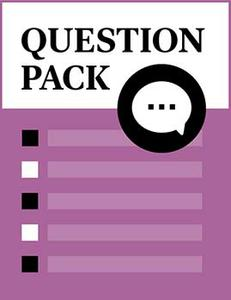# Control Theory

Source: Saylor

Student Price: Free with Top Hat Pro Subscription

A question pack on dynamic systems and controls.

Control Theory Q17

Compensation control techniques use which of the following?

A

B

Phase lag

C

Integration

D

All of the above

Control Theory Q16

Compensation control techniques use which of the following?

A

B

Phase lag

C

Integration

D

All of the above

Control Theory Q15

Controller design using frequency is based on which of the following?

A

Open‑loop time response

B

Closed‑loop time response

C

Open‑loop frequency response

D

Closed‑loop frequency response

Control Theory Q14

If the error signal is ______________, then the output of the integrator stays constant.

A

0.1

B

1

C

2

D

0

Control Theory Q13

In proportional control systems, the measured output is ______________ the input signal to form the error signal.

A

B

Subtracted from

C

Multiplied with

D

Divided by

Control Theory Q12

In proportional control systems, if the sensor gain is ______________, then the output is equal to input.

A

1

B

0.1

C

2

D

0.01

Control Theory Q11

Lead compensation is equivalent to ______________ control?

A

P

B

D

C

PD

D

PID

Control Theory Q10

Many open‑loop systems have high magnitude (gain) at natural frequency equal to ______________.

A

Zero

B

One

C

Infinity

D

Ten

Control Theory Q9

Many open‑loop systems have low magnitude (gain) at natural frequency equal to ______________.

A

Zero

B

One

C

Infinity

D

Ten

Control Theory Q18

The primary objective of lead compensation is to increase ______________ near crossover frequency?

A

Phase margin

B

Gain margin

C

Natural frequency

D

Overshoot

Control Theory Q7

In integral control, which of the following is proportional to the integral of the error?

A

Input

B

Output

C

Control effort

D

Response

Control Theory Q8

In proportional control systems, the control effort is proportional to which of the following signals?

A

Input

B

Measured input

C

Output

D

Error

Control Theory Q5

Pre‑filters are generally used to cancel which of the following?

A

Zeros

B

Poles

C

Gain

D

Phase

Control Theory Q6

The accuracy of proportional control is determined by which of the following?

A

B

Phase margin

C

Open loop gain

D

Closed loop gain

Control Theory Q4

Control systems generally consist of which of the following?

A

Input

B

System

C

Output

D

All of the above

Control Theory Q2

For pole‑zero cancellation which of the following candidate controllers is generally used?

A

P controller

B

PD controller

C

PI controller

D

PID controller

Control Theory Q3

For strategic zero placement, which of the following candidate controllers is generally used?

A

P controller

B

PD controller

C

PI controller

D

PID controller

Control Theory Q1

Which of the following regarding robust control systems is false?

A

Robust control systems have high sensitivities.

B

Robust control systems have low sensitivities.

C

Robust control is stable over wide range of parameter variations.

D

All of the above

CC BY 3.0 - Saylor